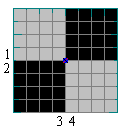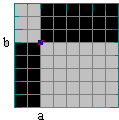# Changing Colors, an Interactive Activity

In the applet below, clicking on a button (triangles on the sides of the board) swaps board colors either in a whole row or in a whole column. Is it possible to have all squares but one white?

Experiment with the applet before looking for a solution.

### This applet requires Sun's Java VM 2 which your browser may perceive as a popup. Which it is not. If you want to see the applet work, visit Sun's website at https://www.java.com/en/download/index.jsp, download and install Java VM and enjoy the applet.

 What if applet does not run?## Solution

Let n×n be the size of the board. For n even, there is a simple solution. Assume we would like to change colors in a row (or a column) with a black and b white squares. Obviously, a + b = n. After the move there will be b black squares. The difference in the number of black squares before and after the move is a - b = a - (n - a) = 2a - n. Thus, if n is even, we'll always end up with an even number of black squares. Therefore, it's impossible to make all but one square white.

What we did was finding an invariant (i.e., a quantity that does not change) under any of legal moves. It's the parity of the number of black squares. For even n, this number always stays even. It's clear that when n is odd the parity changes with every move. So, originally I thought that for odd n the puzzle is easily solvable. After some experimentation, I am now convinced that it is not. I am also pretty sure it's again a matter of finding an invariant quantity. Is it?. What about (a-b)?

I also believe that stronger results may be proven for both n even and odd. Obviously, the set of legal moves in the puzzle is much weaker than that in the Magic Squares or 4×4 coloring problem. When you experiment with the puzzle you can't help noticing that only a limited number of patterns ever appear as a result of your actions. Can you characterize these patterns?

## Solution, Part 2

The puzzle is not very sophisticated. After playing with it awhile I have observed that some quantities of black squares never come up. One can't get 1 black square, but also I never succeeded in getting 2, 3 if n>3, or 4 if n>4, etc. squares. Also the emerging patterns seemed frequently to repeat themselves in a monotonous manner. At this point I tried to classify patterns.

First of all, note that number of black and white squares stays invariant after swapping any two rows or any two columns. Furthermore, consider two configurations in which, say, two columns have been swapped, but otherwise identical. There is an important observation:

After an arbitrary sequence of moves applied to the two configurations, the number of black squares in both will be identical.

The fact bears a rigorous proof. Since the two configurations have identical columns, column moves really do not introduce additional differences. In each row, two squares have been swapped, but otherwise configurations are identical. Therefore, row operations too do not change the relative number of black squares.

Now, let's say that two configurations are equivalent if

1. they have the same number of black squares, and
2. no legal move applied to both configurations changes the fact #1.We just saw that swapping two columns (or two rows) results in an equivalent configuration. By the same token, swapping columns and/or rows repeatedly generates a sequence of equivalent configurations. Thus, the starting configuration is equivalent to the one on right. The center point of the grid splits the board into four regions. Actually, every configuration is equivalent to a similar four region one (can you establish this?)The four region configurations differ by the location of the center of the cross that splits the board. The legal moves may be thought of as changing its location. For example, changing colors in row 1 moves the spot one node up. Moves on the row 2, columns 3 or 4 move it down, left, and right, respectively. Let a and b be coordinates of the center of the cross. Then the number of black squares is defined by the following function:

f(a,b) = a(n-b) + b(n-a)

The function has two symmetric properties: f(a,b) = f(n-a,n-b) and f(a,b) = f(b,a) which help save some effort when trying to list its values. For small values of n it's quite feasible to list all possible values of f for both a and b changing between 0 and n. For example, if n=3 we have:

f(0,0) = 0, f(0,1) = 3, f(0,2) = 6, f(0,3) = 9, f(1,1) = 4, f(1,2) = 5

No other values are possible; for

f(1,0) = f(0,1), f(1,3) = f(2,0) = f(0,2), f(2,1) = f(1,2), f(2,2) = f(1,1), f(2,3) = f(1,0), etc.

It appears that, for every n, no configuration may actually have the number of black squares between 0 and n, 1 in particular. Do you see a general proof of this result?

Richard Beigel gave a complete answer to that question.

(Assume one is allowed to change colors in the cells parallel to one of the diagonals. Even with so much freedom, not all color distributions of cells could be reached from a given starting configuration. There is an additional applet to experiment with.)• Chessboard
• Plus or Minus Game
• Solitaire on a Circle
• Squares, Circles, and Triangles
• Calendar Magic
• Counting Diagonals in a Convex Polygon
• The Game of Fif
• Nim
•